# 959. Regions Cut By Slashes 由斜杠划分区域

@TOC

## # 题目描述

In a N x N `grid` composed of 1 x 1 squares, each 1 x 1 square consists of a `/`, `\`, or blank space. These characters divide the square into contiguous regions.

(Note that backslash characters are escaped, so a `\` is represented as `"\\"`.)

Return the number of regions.

Example 1:

``````Input:
[
" /",
"/ "
]
Output: 2
Explanation: The 2x2 grid is as follows:
``````Example 2:

``````Input:
[
" /",
"  "
]
Output: 1
Explanation: The 2x2 grid is as follows:
``````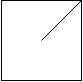Example 3:

``````Input:
[
"\\/",
"/\\"
]
Output: 4
Explanation: (Recall that because \ characters are escaped, "\\/" refers to \/, and "/\\" refers to /\.)
The 2x2 grid is as follows:
``````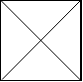Example 4:

``````Input:
[
"/\\",
"\\/"
]
Output: 5
Explanation: (Recall that because \ characters are escaped, "/\\" refers to /\, and "\\/" refers to \/.)
The 2x2 grid is as follows:
``````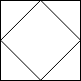Example 5:

``````Input:
[
"//",
"/ "
]
Output: 3
Explanation: The 2x2 grid is as follows:
``````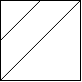Note:

1. `1 <= grid.length == grid.length <= 30`
2. `grid[i][j]` is either `'/'`, `'\'`, or `' '`.

## # 题目大意

``````[
"/\\",
"\\/"
]
``````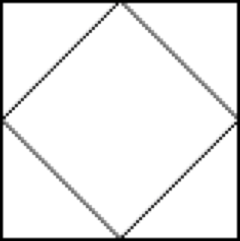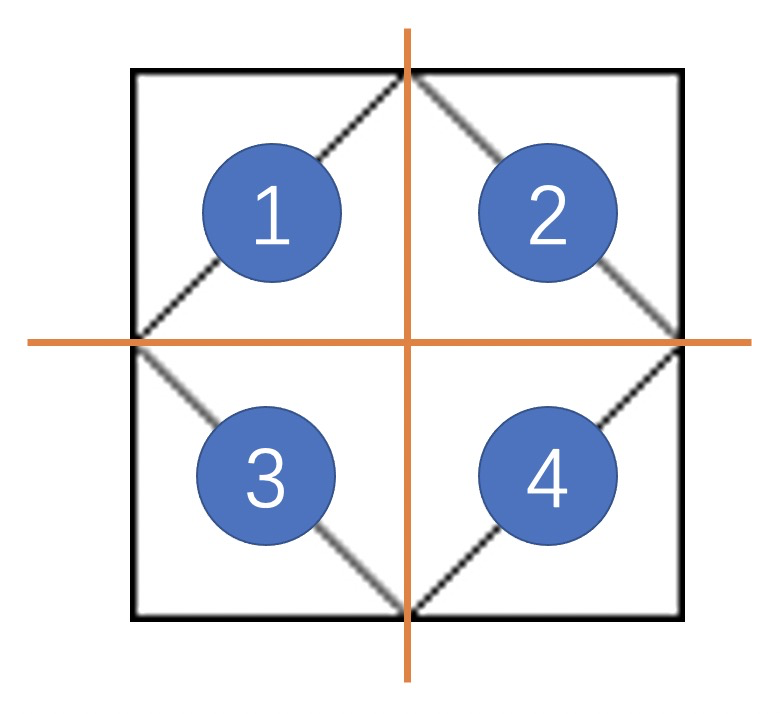## # 解题思路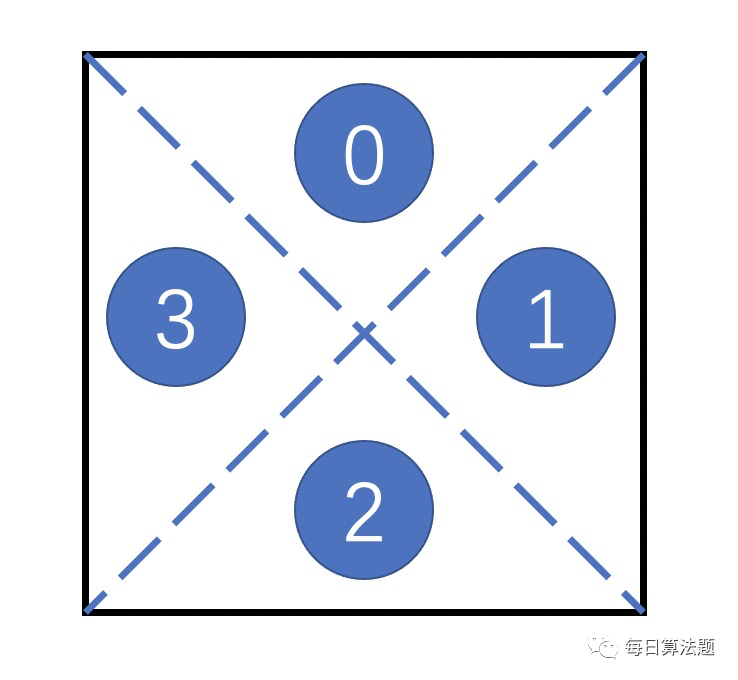1. 左右相邻的两个格子，是左边格子的 ① 和右边格子的 ③ 连通。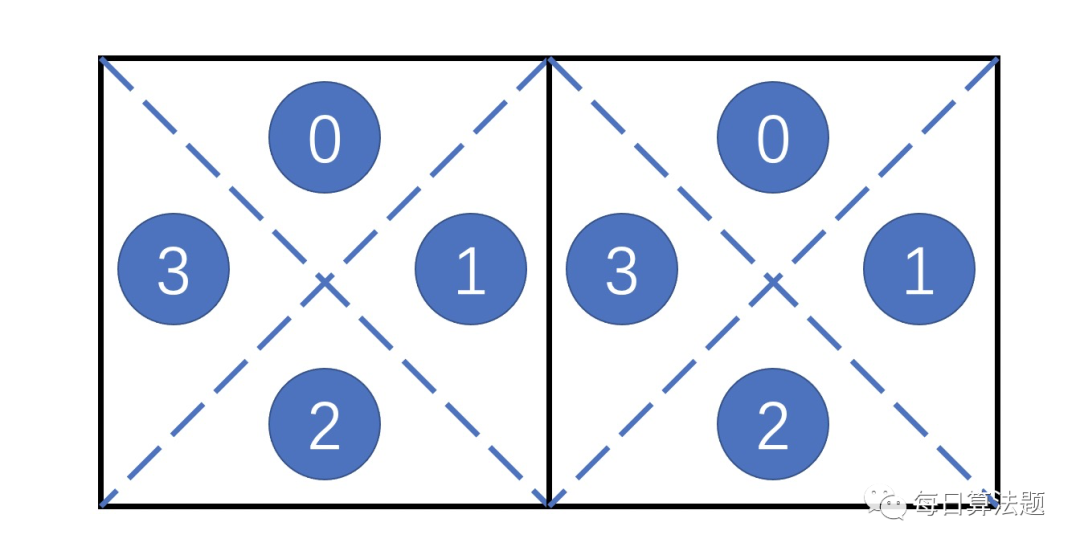1. 上下相邻的两个格子，是上边格子的 ② 和下边格子的 0️⃣ 相邻。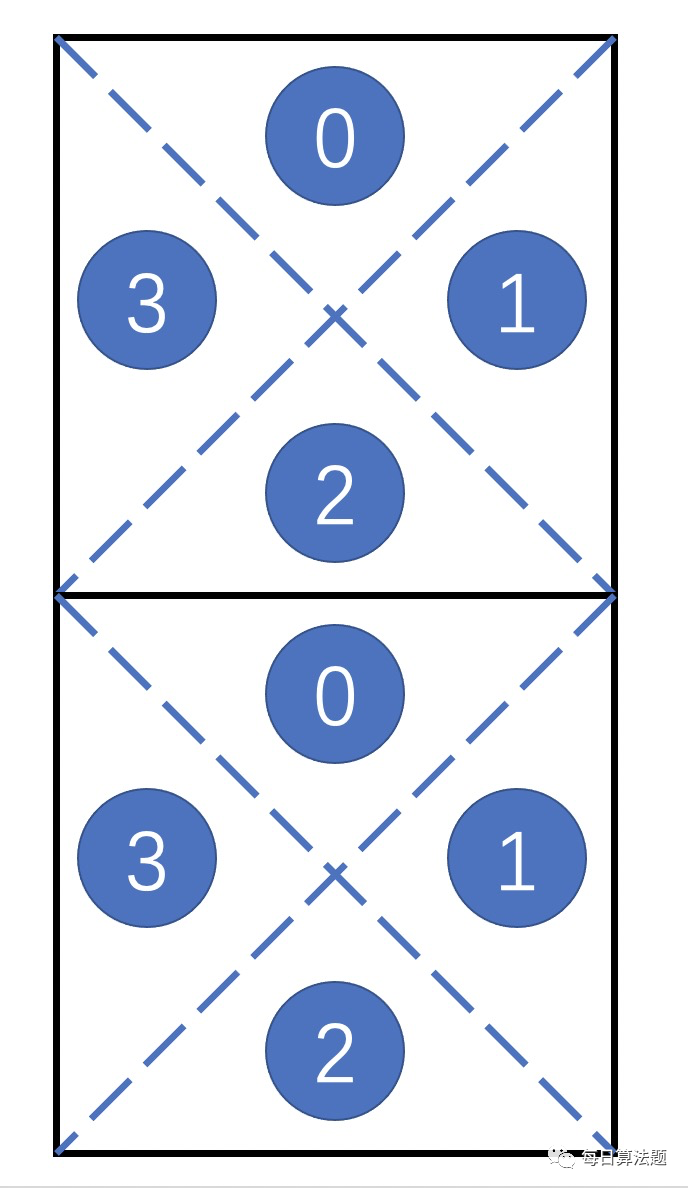## # 代码

• 如果当前的行 row > 0，这个格子的 0️⃣ 需要跟它上面格子的 ② 连通；
• 如果当前的列 col > 0，这个格子的 ③ 需要跟它左边格子的 ① 连通。
• 如果当前格子的状态是 "/"，则当前格子的 0️⃣ 和 ③ 连通、① 和 ② 连通；
• 如果当前格子的状态是 "\"，即对应了下图的 0️⃣ 和 ① 连通、③ 和 ② 连通；
• 如果格子内没有线，则 4 个区域都连通。

``````class Solution(object):
def regionsBySlashes(self, grid):
"""
:type grid: List[str]
:rtype: int
"""
self.N = len(grid)
m_ = range(self.N * self.N * 4)
self.count = self.N * self.N * 4
for row in range(self.N):
line = grid[row]
for col in range(self.N):
w = line[col]
if row > 0:
self.union(m_, self.position(row - 1, col, 2), self.position(row, col, 0))
if col > 0:
self.union(m_, self.position(row, col - 1, 1), self.position(row, col, 3))
if w != '/':
self.union(m_, self.position(row, col, 0), self.position(row, col, 1))
self.union(m_, self.position(row, col, 3), self.position(row, col, 2))
if w != '\\':
self.union(m_, self.position(row, col, 0), self.position(row, col, 3))
self.union(m_, self.position(row, col, 1), self.position(row, col, 2))
return self.count

def find(self, m_, a):
if m_[a] == a:
return a
return self.find(m_, m_[a])

def union(self, m_, a, b):
pa = self.find(m_, a)
pb = self.find(m_, b)
if (pa == pb):
return
m_[pa] = pb
self.count -= 1

def position(self, r, c, i):
return (r * self.N + c) * 4 + i
``````

## # 日期

2018 年 12 月 16 日 —— 周赛好难 2021 年 1 月 25 日 —— 重写刷了这个题，并推送了微信公众号